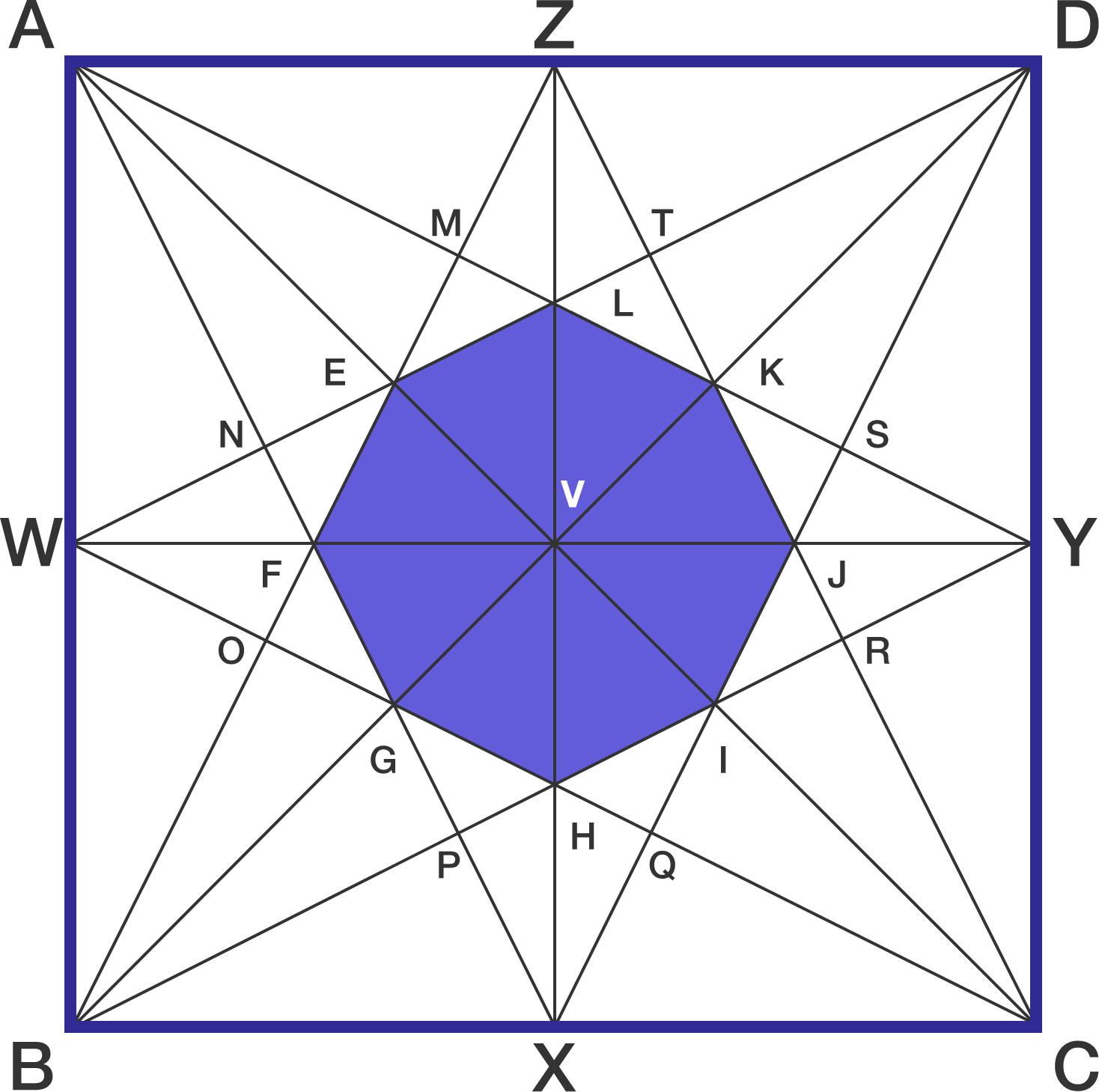# I used 25 alphabets

Geometry Level 2Above figure shows a unit square $ABCD$.

If the area of the octagon $EFGHIJKL$ (in blue) can be expressed as $\dfrac{1}{a}$ , find $a$.

×